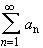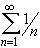#Interactive Real Analysis

Next | Previous | Glossary | Map

## 4.2. Convergence Tests

### Root Test

Consider the series. Then:
• if lim sup | a n | 1/n < 1 then the series converges absolutely
• if lim sup | a n | 1/n > 1 then the series diverges
• if lim sup | a n | 1/n = 1, this test gives no information
Compare this test with the Ratio test. Although this root test is more difficult to apply, it is better than the ratio test in the following sense: there are series for which the ratio test give no information, yet the root test will be conclusive. You can also use the root test to prove the ratio test , but not visa versa.

It is important to remember that when the root test gives 1 as the answer for the lim sup, then no conclusion at all is possible.

The use of the lim sup rather than the regular limit has the advantage that we do not have to be concerned with the existence of a limit. On the other hand, if the regular limit exists, it is the same as the lim sup, so that we are not giving up anything using the lim sup.

 Examples 4.2.14:Does the root test apply to? Does the series converge or diverge ?Does the root test apply to? Does the series converge or diverge ?Does the seriesconverge or diverge ?

Proof:

Assume that lim sup | a n | 1/n < 1: Because of the properties of the limit superior, we know that there exists> 0 and N > 1 such that

| a n | 1/n < 1 -for n > N. Raising both sides to the n-th power we have:
| a n | < (1 -) n
for n > N. But the terms on the right hand side form a convergent geometric series. Hence, by the comparison test the series with terms on the left-hand side will converge absolutely.

The proof for the second case if left as an exercise.

Next | Previous | Glossary | Map